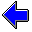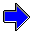Java Security Settings:
This web page employs Java, which requires specific security settings for correct operation.
If the applets on this page do not run correctly, consult the Virtual Chemistry Experiments FAQ
or the Physlet Physics web site for establishing the correct security settings.

# Calorimetry

## Heat of Neutralization

### Concepts

Large concentrations of hydrogen ion and hydroxide ion cannot coexist in solution, because the neutralization reaction

H+ (aq) + OH- (aq)   →   H2O (l)

strongly favors the product (water).

In this experiment, an aqueous hydrochloric acid solution will be added to an aqueous sodium hydroxide solution. The neutralization reaction will occur until either H+ or OH- is entirely consumed. The reactant which is consumed first is called the limiting reactant.

The molar enthalpy of neutralization is defined as

 ΔHneut = qneutn

where qneut is the heat of neutralization, measured calorimetrically, and n is the moles of the limiting reactant.

### Experiment

Objective:

• Determine the molar enthalpy of neutralization.

Approach:

• Add a known volume of 3.00 M aqueous HCl to a known volume of 1.00 M aqueous NaOH.
• Observe the temperature of the system before and after the neutralization reaction occurs.
• Calculate the change in temperature for the system.
• Use the temperature change and heat capacities for the calorimeter and aqueous solution to calculate the heat of neutralization.
• Divide the heat of neutralization by the moles of the limiting reactant to determine the molar enthalpy of neutralization.

### Part 1

In this part of the experiment, the calorimeter is filled with 50.0 mL of 1.00 M aqueous NaOH.
The reservoir contains 20.0 mL of 3.00 M aqueous HCl. Both solutions start at the same initial temperature.

The heat capacity of the calorimeter (Ccal) is 78.2 J oC-1.

The specific heat capacity of the aqueous solutions is 4.184 J oC-1 g-1.

### Part 2

The conditions in this part of the experiment are the same as those in Part 1.

The upper reservoir contains 3.00 M aqueous HCl and the calorimeter initially contains 1.00 M aqueous NaOH. Both solutions start at the same initial temperature.

The heat capacity of the calorimeter (Ccal) is 78.2 J oC-1.

The specific heat capacity of the aqueous solutions is 4.184 J oC-1 g-1.

Repeat the experiment using various volumes of HCl and NaOH. You should get the same molar enthalpy of neutralization.

Volume of
3.00 M HCl
mL

Volume of
1.00 M NaOH
mLHeat of Solution of Ammonium Nitrate                     Heat of Solution of Sulfuric AcidCalorimetry Home PageVirtual Chemistry Home Page

HeatOfNeutralization.html version 2.1
© 2000-2014 David N. Blauch